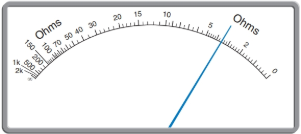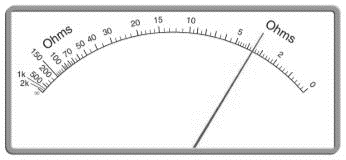Chapter 4, Problem 20T

Chapter
Section
Textbook Problem

# Read each scale:To determine

To calculate: The measurement on scale, provided as,Explanation

Given Information:

Formula used:

Step 1: To determine the whole number find the closest graduation where the needle point.

Step 2: Calculate the value of subdivision and count number of graduation and add them.

Step 3: Add the number from step 1 and step 2.

Calculation:

Consider the scale provided as:

This scale shows a non-uniform scale. Nonuniform scale is defined as scale which is not uniformly spaced. Each subdivision has different quantity.

Range in between 05 Ω

### Still sussing out bartleby?

Check out a sample textbook solution.

See a sample solution

#### The Solution to Your Study Problems

Bartleby provides explanations to thousands of textbook problems written by our experts, many with advanced degrees!

Get Started

## Additional Math Solutions

#### Find more solutions based on key concepts